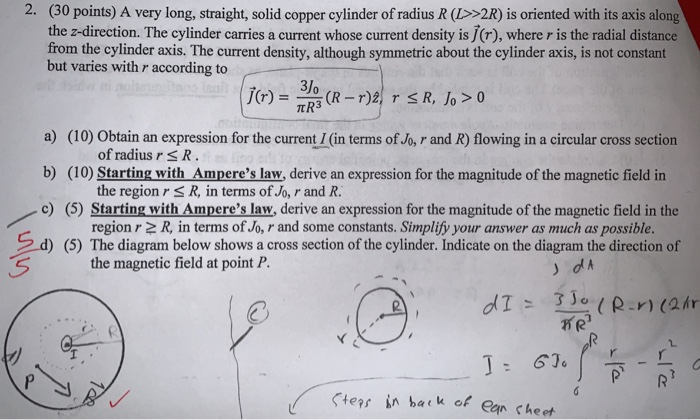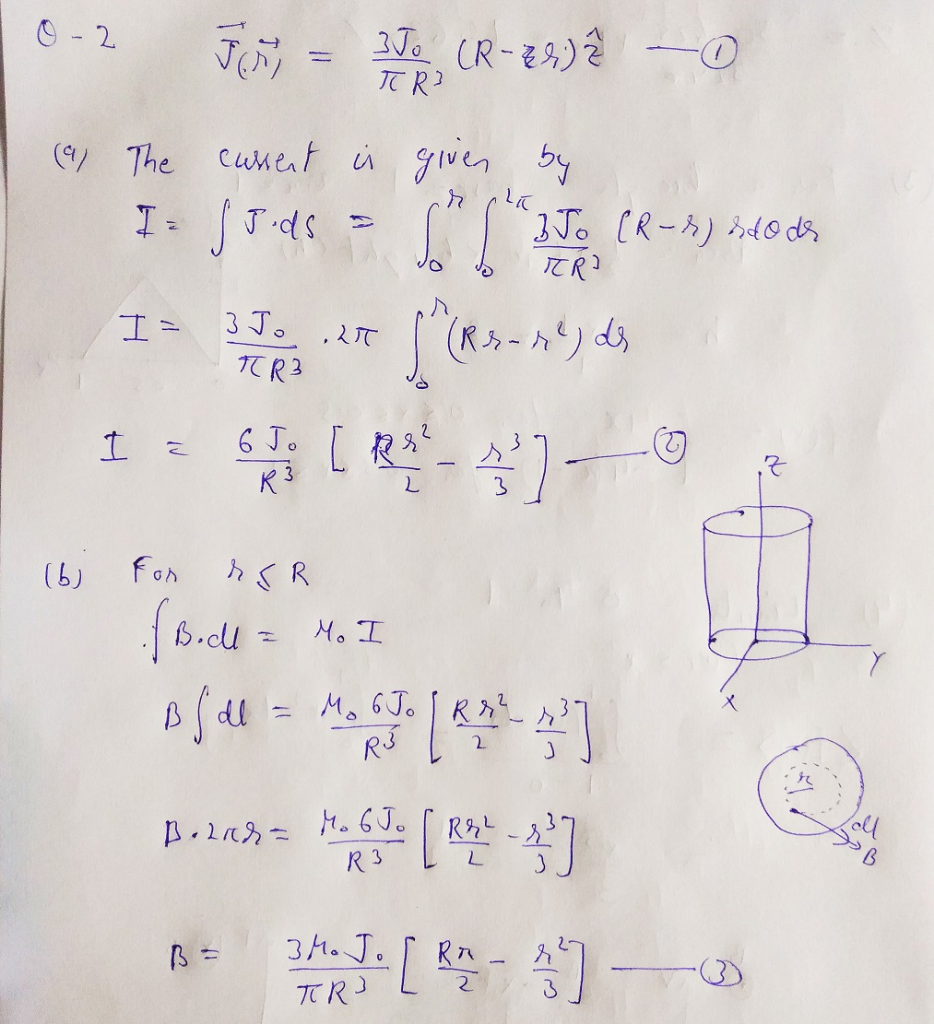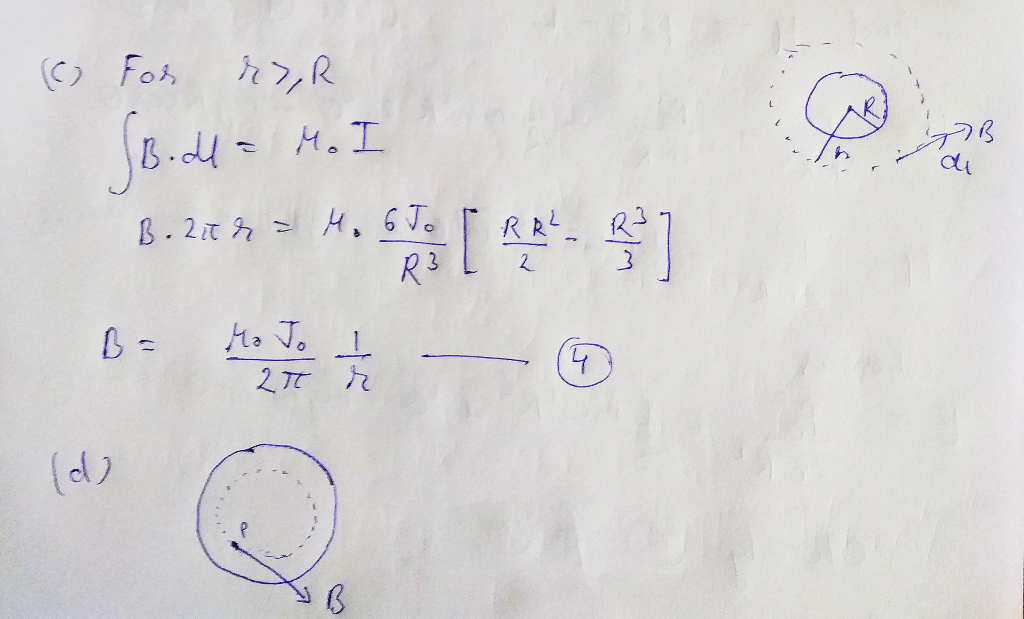Homework Help Question & Answers

2. (30 points) A very long, straight, solid copper cylinder of radius R (>2R) is oriented with it...2. (30 points) A very long, straight, solid copper cylinder of radius R (>2R) is oriented with its axis along e z-direction. The cylinder carries a current whose current density is j(r), where r is the radial distance from the cylinder axis. The current density, although symmetric about the cylinder axis, is not constant but varies with r according to 31o a) (10) Obtain an expression for the current /(in terms of Jo, r and R) flowing in a circular cross section b) (10) Starting with Ampere's law, derive an expression for the magnitude of the magnetic field in c) (5) Starting with Ampere's law, derive an expression for the magnitude of the magnetic field in the 2d) (5) The diagram below shows a cross section of the cylinder. Indicate on the diagram the direction of of radius rsR the region rS R, in terms of Jo, r and R. region r 2 R, in terms of Jo, r and some constants. Simplify your answer as much as possible. Sthe magnetic field at point P 了Jo Sters in ba o ean cheehAdd Answer of: 2. (30 points) A very long, straight, solid copper cylinder of radius R (>2R) is oriented with it...
More Homework Help Questions Additional questions in this topic.

• (Note: In this problem, a "cylinder means the can-shaped figure that we used to recognize as a cylinder not the cylinder defined in the sense as in Section 12.6.) Problem: Three circular cylinder...

Need Online Homework Help?

Get FREE EXPERT Answers
WITHIN MINUTES
Related Questions